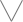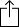C
D
E
F
G
A
B
Chord
Scale
Custom
3nps
CAGED
None
Notes
Degrees
Intervals
None

1
2
3
4
5
6
7
1
2
3
4
5
6
7
8
9
10
11
12
13
14
15
16
17
18
19
20
21
22
23
24
E
B
G
D
A
E
F
G
G
A
A
B
B
C
D
D
E
E
F
G
G
A
A
B
B
C
D
D
E
E
C
D
D
E
E
F
G
G
A
A
B
B
C
D
D
E
E
F
G
G
A
A
B
B
A
A
B
B
C
D
D
E
E
F
G
G
A
A
B
B
C
D
D
E
E
F
G
G
E
E
F
G
G
A
A
B
B
C
D
D
E
E
F
G
G
A
A
B
B
C
D
D
B
B
C
D
D
E
E
F
G
G
A
A
B
B
C
D
D
E
E
F
G
G
A
A
F
G
G
A
A
B
B
C
D
D
E
E
F
G
G
A
A
B
B
C
D
D
E
E

G Minor 13th for Guitar - 3nps Chart

A G Minor 13th chord, also written as Gm13, G-13, Gmin13 or GMI13 contains the notes G, Bb, D, F, A, C and E. The chord is comprised of the intervals m3, M3, m3, M3, m3 and M3.

G
B
D
F
A
C
Em3
M3
m3
M3
m3
M3
Play scaleand then